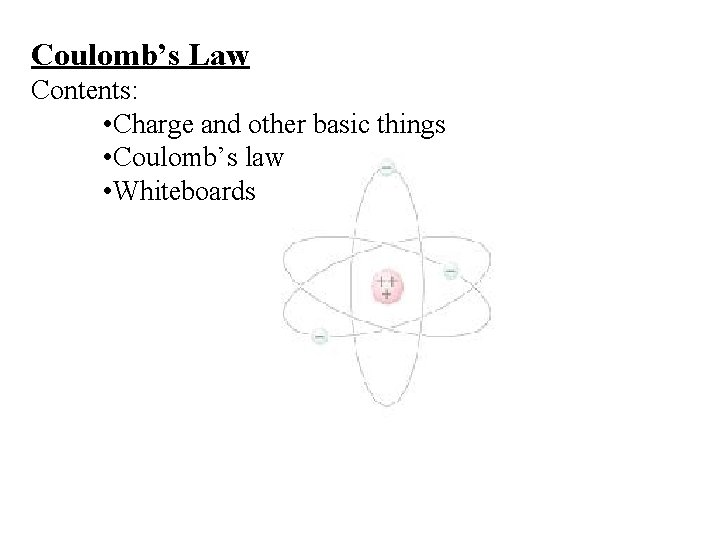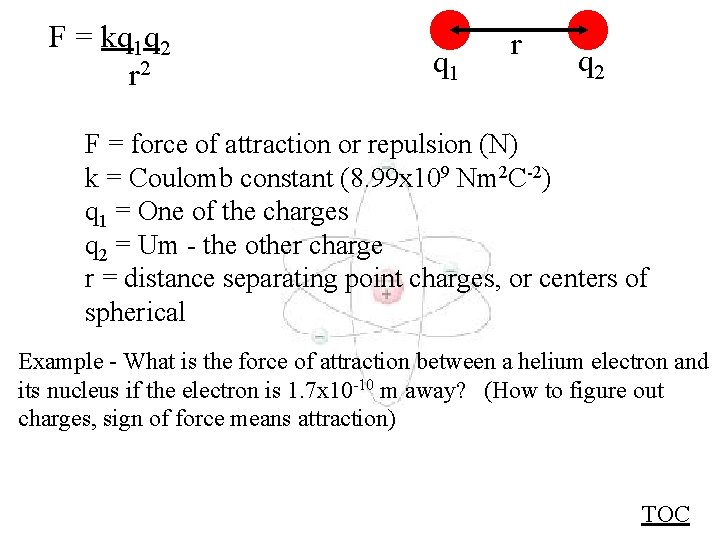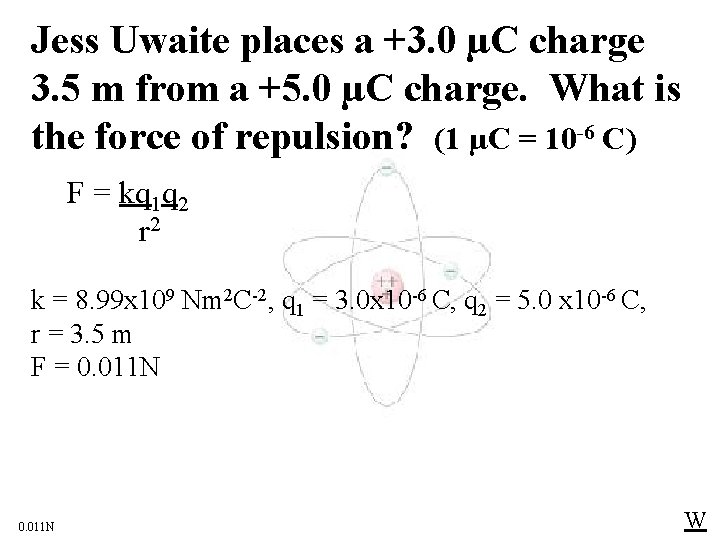# Coulombs Law Contents Charge and other basic things

• Slides: 10Coulomb’s Law Contents: • Charge and other basic things • Coulomb’s law • WhiteboardsBasic Stuff • Charge is in Coulombs (C) (1 C = 1 A·s) • Signed quantity (+/-) • e = 1. 602 x 10 -19 C • Protons are +, electrons are • 1 C = 6. 25 x 1018 electrons or protons • 1 C = 10 -6 C • Charge is conserved • Likes repel, opposites attract • Demos: pie plates, 2 balloons, hair, bubbles, rice crispies TOCCoulomb’s Law Just like gravity: F = kq 1 q 2 r 2 q 1 r q 2 F = force of attraction or repulsion (N) k = Coulomb constant (8. 99 x 109 Nm 2 C-2) q 1 = charge 1 (C) q 2 = charge 2 (C) r = center to center distance (m) TOCF = kq 1 q 2 r 2 q 1 r q 2 F = force of attraction or repulsion (N) k = Coulomb constant (8. 99 x 109 Nm 2 C-2) q 1 = One of the charges q 2 = Um - the other charge r = distance separating point charges, or centers of spherical Example - What is the force of attraction between a helium electron and its nucleus if the electron is 1. 7 x 10 -10 m away? (How to figure out charges, sign of force means attraction) TOCWhiteboards: Coulomb’s Law 1|2|3|4 TOCJess Uwaite places a +3. 0 µC charge 3. 5 m from a +5. 0 µC charge. What is the force of repulsion? (1 µC = 10 -6 C) F = kq 1 q 2 r 2 k = 8. 99 x 109 Nm 2 C-2, q 1 = 3. 0 x 10 -6 C, q 2 = 5. 0 x 10 -6 C, r = 3. 5 m F = 0. 011 N WNoah Verkreinatlaad places a 5. 0 C charge how far from a 3. 0 C charge to make the force between them exactly 4. 00 N? F = kq 1 q 2 r 2 k = 8. 99 x 109 Nm 2 C-2, q 1 = 5. 0 C, q 2 = 3. 0 C, F = 4. 0 N r = 1. 8 x 105 m = 180 km = 100 miles wow 1. 8 x 105 m or 180 km WHughe Jass notices that there is an attractive force of 1. 3 n. N (Nano Newtons 10 -9 N) on an electron from another charge that is 42 nm (42 x 10 -9 m) away. What is that other charge? F = kq 1 q 2 r 2 k = 8. 99 x 109 Nm 2 C-2, q 1 = -1. 602 x 10 -19 C, F = -1. 3 x 10 -9 N r = 42 x 10 -9 m q 2 = 1. 6 x 10 -15 C WIdo Wanamaker places an electron 1. 32 x 10 -10 m from a proton. What is the force of attraction? F = kq 1 q 2 r 2 k = 8. 99 x 109 Nm 2 C-2, q 1 = -1. 602 x 10 -19 C, q 2 = +1. 602 x 10 -19 C, r = 1. 32 x 10 -10 m F = -1. 32 x 10 -8 N WInsulators and Conductors Insulators - Charge cannot move Conductors - Charge moves freely - stays on outside Insulators Glass Wood Plastics (organics) Dry Air (10, 000 V/inch) Vacuum Pure water Conductors Metals - sea of electrons Graphite Semiconductors Salt water Any ionic solutions Ionized air TOC